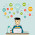## Tuesday, July 21, 2009

### Geometric and harmonic means in R

Compute the geometric mean and harmonic mean in R of this sequence.

10, 2, 19, 24, 6, 23, 47, 24, 54, 77

These features are not present in the standard package of R, although they are easily available in some packets.
However, it is easy to calculate these values simply by remembering the mathematical formulas, and applying them in R.

$$H = \frac{n}{\frac{1}{x_1} + \frac{1}{x_2} + \cdots + \frac{1}{x_n}} = \frac{n}{\sum_{i=1}^n \frac{1}{x_i}}, \qquad x_i > 0 \text{ for all } i.$$

In R language:

a = c(10, 2, 19, 24, 6, 23, 47, 24, 54, 77)1/mean(1/a) #compute the harmonic mean 10.01109

$$\overline{a}_{geom}=\bigg(\prod_{i=1}^n a_i \bigg)^{1/n} = \sqrt[n]{a_1 \cdot a_2 \cdots a_n}$$

In R language

a = c(10, 2, 19, 24, 6, 23, 47, 24, 54, 77)n = length(a) #now n is equal to the number of elements in aprod(a)^(1/n) #compute the geometric mean 18.92809

1.2.